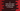# C program to read user input and store them in two dimensional array### C program to read user input strings and store them in a two dimensional array :

In this tutorial, we will learn how to read few user input strings and store them in a two dimensional array. To understand how two dimensional array stores string, let’s take a look at the image below :You can think it like an array with each element can contain one string. Each element is also an array of characters. The first row contains one string APPLE. A is stored in the 0th position, P is in 1st position etc. Similarly, the first row contains MANGO etc. So, our aim is to write one program in C that will ask the user to enter string for each row of the array and then it will store these strings in the array. A two dimensional array is denoted as arrayName[row][column] , where row is the total row count and column is the total column count of the array.

### C program :

``````#include <stdio.h>

int main(){
//1
int count = 3;
int i;

//2
char userInputString[count];

//3
for(i = 0 ; i < count ; i++){
//4
printf("Enter string %d : ",i);
fgets(userInputString[i],100,stdin);
}

//5
printf("You have entered : \n");
for(i = 0 ; i < count ; i++){
//6
printf("%s",userInputString[i]);
}

return 0;

}``````

### Explanation :

The commented numbers in the above program denote the step number below :

1. Create one integer count to store the total number of strings to store. Integer i is for use in loops.
2. Create one two dimensional array. This array can store count number of strings. The maximum size of each string is 100.
3. Run one for loop to read user input strings.
4. Ask the user to enter a string. Read it and store it in the two dimensional array.
5. Print out all the strings the user has just entered.
6. Run one for loop again, and print out the contents of each index of the two dimensional array.

### Sample Output :

``````Enter string 0 : Hello
Enter string 1 : world
Enter string 2 : !!
You have entered :
Hello
world
!!

Enter string 0 : apple
Enter string 1 : mango
Enter string 2 : banana
You have entered :
apple
mango
banana``````